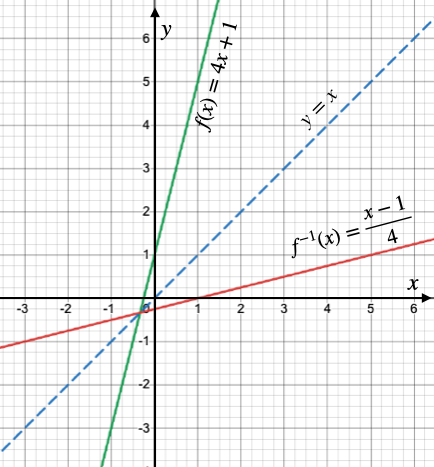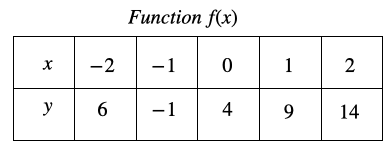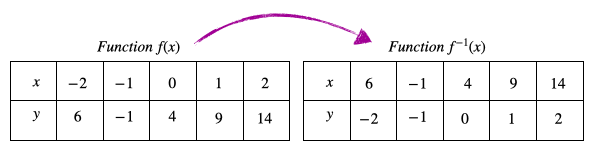# Algebra 1 - Inverse Functions

## Introduction

• Inverse functions exist only for one-to-one functions.
• Recall that a function is one-to-one if and only if its graph passes the horizontal line test.
• Given two one-to-one functions $f\left(x\right)$ and $g\left(x\right)$ if $\left(f\circ g\right)\left(x\right)=x$ AND $\left(g\circ f\right)\left(x\right)=x$, then we can say that $f\left(x\right)$ and $g\left(x\right)$ are inverses of each other and we denote it by ${f}^{-1}\left(x\right)=g\left(x\right)$ and ${g}^{-1}\left(x\right)=f\left(x\right)$.
• The domain of an inverse function ${f}^{-1}$ is the range of the function $f$ and vice-versa.

## Graphs of Inverse Functions

• Let us analyze the graph of $f\left(x\right)=4x+1$ and its inverse ${f}^{-1}\left(x\right)=\frac{x-1}{4}$ shown below.• In the figure shown above, we can see that both curves (function & its inverse) are mirror images of each other with respect to the line $y=x$.
• Thus, we can conclude that the graph of a function $\left(f\right)$ and its inverse $\left({f}^{-1}\right)$ are symmetric across the line $y=x$.

## Finding the Inverse of a Function

• Follow these steps to find the inverse of a function:
• Replace $f\left(x\right)$ with y.
• Switch or swap the position of x & y.
• Solve for y.
• The solution for y will give you ${f}^{-1}\left(x\right)$

## Solved Examples

Question 1: Find the inverse of the function represented by the table of values below.Solution: We know that the domain of ${f}^{-1}$ is the range of $f$ and the range of ${f}^{-1}$ is the domain of $f$.

Hence, the inverse of the given function is shown in the table below.Question 2: What is the inverse of the function $f\left(x\right)=3x+7$?

Solution: $f\left(x\right)=3x+7$

Replace $f\left(x\right)$ with $y$.

$y=3x+7$

Swap the position of .

$x=3y+7$

Solve for $y$.

$3y=x-7$

$y=\frac{x-7}{3}$

${f}^{-1}\left(x\right)=\frac{x-7}{3}$

Question 3: What is the inverse of the function $f\left(x\right)=\frac{3x}{2x-5}$? Determine the domain and range of its inverse.

Solution: $f\left(x\right)=\frac{3x}{2x-5}$

Replace $f\left(x\right)$ with $y$.

$y=\frac{3x}{2x-5}$

Swap the position of .

$x=\frac{3y}{2y-5}$

Solve for $y$.

$2xy-5x=3y$

$2xy-3y=5x$

$\left(2x-3\right)y=5x$

$y=\frac{5x}{\left(2x-3\right)}$

${f}^{-1}\left(x\right)=\frac{5x}{\left(2x-3\right)}$

The domain and range of ${f}^{-1}\left(x\right)$ are as follows: Domain:  and Range:

Question 4: What is the inverse of the function $y={x}^{2}-4x-5?$

Solution: The given function is NOT one-to-one. Thus, we need to restrict the domain in order for the function and its inverse to become one-to-one.

The domain of this function should be either .

$f\left(x\right)={x}^{2}-4x-5$

Replace $f\left(x\right)$ with $y$.

$y={x}^{2}-4x-5$

Swap the position of .

$x={y}^{2}-4y-5$

Solve for $y$.

${y}^{2}-4y-5-x=0$

${y}^{2}-4y-\left(5+x\right)=0$

$y=\frac{-b±\sqrt{{b}^{2}-4ac}}{2a}$

$y=\frac{-\left(-4\right)±\sqrt{{\left(-4\right)}^{2}-4×\left(1\right)×\left\{-\left(5+x\right)\right\}}}{2×\left(1\right)}$

$y=\frac{4±\sqrt{16+4\left(5+x\right)}}{2}$

$y=\frac{4±\sqrt{16+20+4x}}{2}$

$y=\frac{4±\sqrt{36+4x}}{2}$

$y=\frac{4±2\sqrt{9+x}}{2}$

$y=2±\sqrt{9+x}$

$y=2±\sqrt{9+x}$ is not a function. So we have expressed it as either .

The inverse of is .

The inverse of  is .

## Cheat Sheet

• Inverse functions exist only for one-to-one functions.
• Two functions, $f$ and $g$, are inverses of each other if and only if $f\left[g\left(x\right)\right]=g\left[f\left(x\right)\right]=x$.
• If ${f}^{-1}\left(x\right)$ is the inverse of the function $f\left(x\right)$, then the domain of ${f}^{-1}\left(x\right)$ is the range of the $f\left(x\right)$, and the range of ${f}^{-1}\left(x\right)$ is the domain of $f\left(x\right)$.
• If a function and its inverse are graphed, they will be symmetrical about the line $y=x$.

## Blunder Areas

• There are cases in which the inverse of a function is not a function. In these cases, the domain of the function can be restricted to make the inverse a function.
• There are examples wherein the function and its inverse are the same. For example, the inverse of the function, is ${f}^{-1}\left(x\right)=\frac{1}{x}$.
• ${f}^{-1}$ refers to the "inverse function" and is not equal to $\frac{1}{f}$.